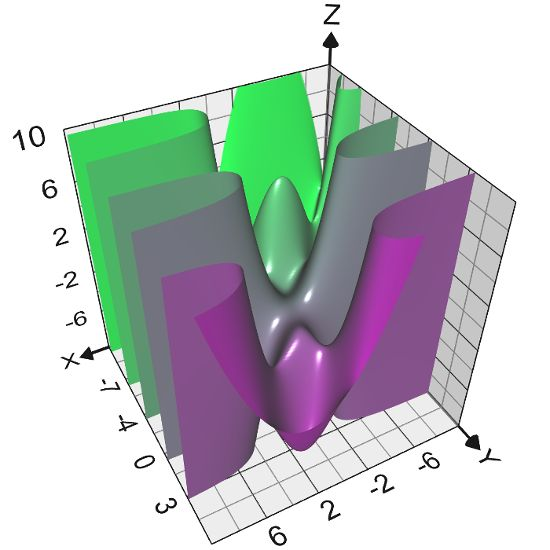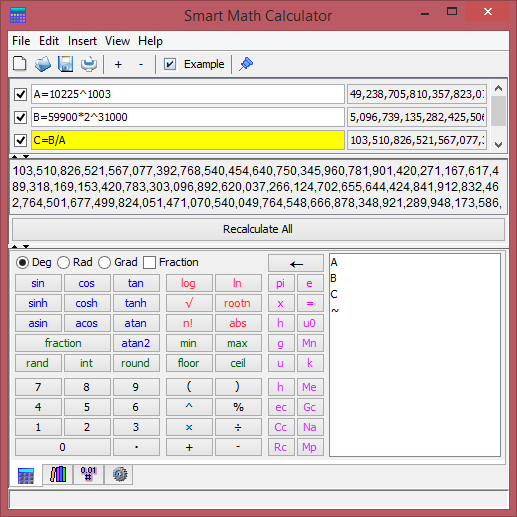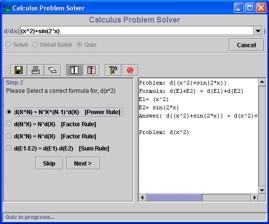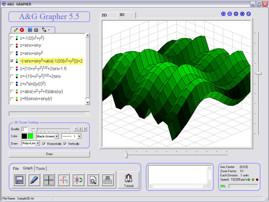﻿ Runiter Math Software Products

# Products

#### Graphing Calculator 3D

Plot equations and scatter points with this powerful graphing software.#### Smart Math Calculator

Compute, edit multiple math expressions and define variables with ease.#### Statistics Problem Solver

Learn basic statistics with this step-by-step problem solver.#### Calculus Problem Solver

Learn differential calculus by solving differentiation problems and view the step-by-step solutions.#### A&G Grapher

A tool for basic plotting needs. Not as powerful as Graphing Calculator 3D but some may prefer this for basic 2D plots.